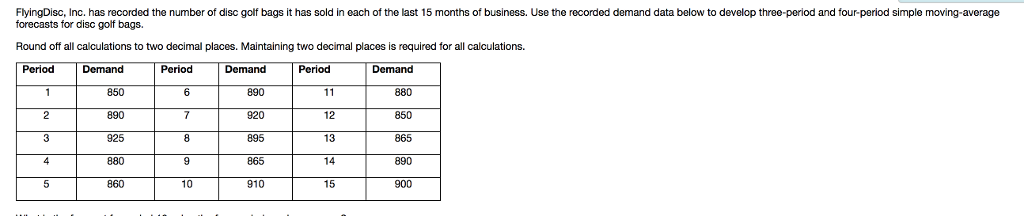# Question & Answer: What is the forecast for period 16 using the four-period moving average? What is the MAD…..

What is the forecast for period 16 using the four-period moving average?

What is the MAD for the three-period moving-average forecast as of period 15?

What is the MAPE for the three-period moving average forecast as of period 15?

Don't use plagiarized sources. Get Your Custom Essay on
Question & Answer: What is the forecast for period 16 using the four-period moving average? What is the MAD…..
GET AN ESSAY WRITTEN FOR YOU FROM AS LOW AS \$13/PAGE

What is the MAD for the four-period moving average forecast as of period 15?

What is the MAPE for the four-period moving average forecast as of period 15?

Which forecast is the best (the three-period or four-period simple moving-average forecast method)?FlyingDisc, Inc. has recorded the number of disc golf bags it has sold in each of the last 15 months of business. Use the recorded demand data below to develop three-period and four-period simple moving-average forecasts for disc golf bags. Round off all calculations to two decimal places. Maintaining two decimal places is required for all calculations. Period Demand Period 890 920 895 865 910 880 850 865 890 890 12 13 14 15 925 10

Following table illustrate and presents relevant data leading to determination of Moving average Forecast , MAD and MAPE

 Period Demand Forecast value – 3 period moving average Absolute deviation ( AD) Absolute Percentage Error ( APE) Forecast value – 4 period moving average Absolute deviation (AD) Absolute Percentage Error ( APE) 1 850 2 890 3 925 4 880 888.33 8.33 0.94 5 860 898.33 38.33 4.27 886.25 26.25 2.96 6 890 888.33 1.67 0.19 888.75 1.25 0.14 7 920 876.67 43.33 4.94 888.75 31.25 3.52 8 895 890.00 5.00 0.56 887.5 7.5 0.85 9 865 901.67 36.67 4.07 891.25 26.25 2.95 10 910 893.33 16.67 1.87 892.5 17.5 1.96 11 880 890.00 10.00 1.12 897.5 17.5 1.95 12 850 885.00 35.00 3.95 887.5 37.5 4.23 13 865 880.00 15.00 1.70 876.25 11.25 1.28 14 890 865.00 25.00 2.89 876.25 13.75 1.57 15 900 868.33 31.67 3.65 871.25 28.75 3.30 Sum = 266.67 30.15 218.75 24.70

Following are to be noted :

a)       Three Period moving average Forecast :

Ft = ( Dt-1 + Dt-2 + Dt-3) / 3

b)      Four period moving average forecast :

Ft =( Dt-1 + Dt-2 + Dt-3 + Dt-4)/4

Ft = Forecast for period t

Dt-1 = Demand for period t-1

Dt-2 = Demand for period t-2

Dt-3 = Demand for period t-3

Dt-4 = demand for period t-4

c)       Thus forecast for period 16 using 4 period moving average = ( 850 + 865 + 890 + 900 ) /4 = 876.25

d)      MAD for three period moving average = Sum of absolute deviations (AD) / 12 = 266.67/12 = 22.22

e)      MAPE for three period moving average = Sum of absolute percentage error / 12 = 30.15/12 = 2.51

f)        MAD for four period moving average = Sum of absolute deviations (AD) /11 = 218.75 / 11 = 19.88

g)       MAPE for four period moving average = Sum of absolute percentage error /11 = 24.70/11 = 2.24

Since both MAD as well as MAPE for four period moving average is less than MAD and MAPE of three period moving average , Four Period moving average is a better forecast